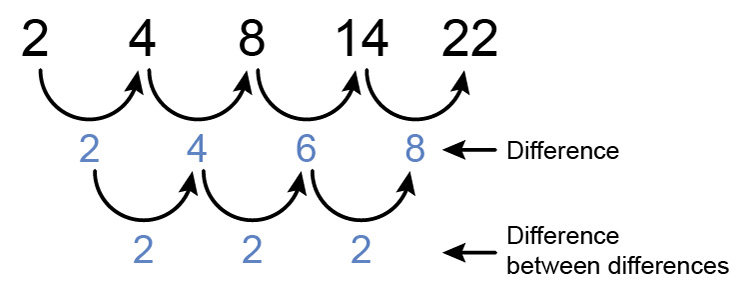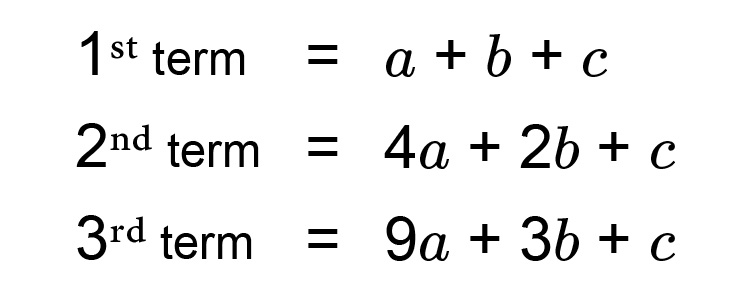# Formula for n^(th) term of a sequence - consistent difference between differences

The formula is an^2+bn+c and this is explained by the following:

The following pattern is known as a quadratic.When we know we are dealing with a sequence that is a quadratic (where the difference between differences are consistent) we must remember the facts about quadratics.See mammoth memory quadratics to understand the above picture.

(A quadratic is an x^2  term =ax^2+bx+c)

ax^2+bx+c

but here the x  is replaced by the letter n  denoting the number in a sequence.and this is all you need to remember because

## The first term is

1st term (where n=1) =atimes1^2+btimes1+c

is the same as

1st term=a+b+c

## The second term is

2nd term (n=2)=atimes2^2+btimes2+c

is the same as

2nd term =4a+2b+c

## The third term is

3rd term (n=3)=atimes3^2+btimes3+c

is the same as

3rd term =9a+3b+c

## Summary

So we knowSo for any quadratic sequence (consistent difference between differences) we can use this information to work out ANY n^(th)  term formula.